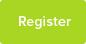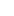# Tutor Hunt Questions

A crowbar is applied such that the fulcrum is 0.05m from the end of the crowbar in contact with the rock. A force of 150 N is applied to the crowbar at a distance of 0.50 M. How much force must be exerted on the rock by the crowbar to move the rock?

The answer is 1500 N but I`m not sure how.
3 years ago

150*0.5 is the moment of the Force applied on the crowbar F*0.05 is the moment exerted on the rock sum of the clockwise moments eqaul sum of anti clockwise moments therefore 150*0.50 = F*0.05 rearrange and calculate: The answer F = 1500N This is the force applied on the rock by the crowbar. as the rock is not moving at first the weight of the will be 1500N. Hope that helped. Zak
04/03/2016 10:05:13 | comment by Zak

Please enter your response to the question below. The student will get a notification as soon your response has been approved by our moderation team.

I personally tend to find it a lot easier visualising an image when it comes to Physics questions! Imagine the image in the link:

www.visualdictionaryonline/images/science/physics-mechanics/lever.jpg

In our scenario..
The "load" is the rock, the "fulcrum" is a fancy word for the pivot and the arrow for effort (on the other side of the fulcrum) is where the force is applied to the crowbar.
It helps to put our information on the diagram!

We also need to know our rules for turning effect of forces:
Rule 1: Moment = Force (N) x Perpendicular distance from pivot (m)
Rule 2: Sum of clockwise moments = sum of anticlockwise moments

It says "A force of 150 N is applied to the crowbar at a distance of 0.50 M." - Moment of the Force force applied on the crowbar (the effort part in our image) is therefore:
150N (Force) x 0.5m (distance) = 75Nm

In the opposite direction we have the force exerted on the rock (our load). We know that the rock is 0.05m away from the fulcrum. But we don`t know the FORCE (N) - which is the part we`re figuring out! to write this down:

??N (Force) x 0.05m (distance) = ?

Rule 2 says opposite direction are equal:
150N (Force) x 0.5m (distance) = ??N (Force) x 0.05m (distance)..
75Nm = ??N x 0.05m..
75Nm/0.05m = 1500N :)
Answered by Amisha | 3 years ago
It is at ninety degrees. Any other angle would involve a components of the force pushing the lever over the pivot point rather than lifting the load.
09/03/2016 12:26:56 | comment by Steven
Think of a see-saw with a heavy man on one end and a small boy on the other. Their respective turning moments are given by Force x distance from the hinge on one side and force x distance on the other, or F1 x D1 = F2 x D2

You have F1, D1 & D2 so just rearrange the equation to give F2. So

F2 = (F1 x D1)/D2 = (150N x 0.5m ) / 0.05m = 1500N.

Which is why the heavy man can balance the see saw with the small boy as long as he sits nearer the hinge (or fulcrum if you want to be posh) :-)
The formula for moments is (Moment = Force * Distance)
However there is a fulcrum involved in this question so we need to do the following:

We know that in a fulcrum, the sum of the clockwise moment is equal to the sum of the anticlockwise moment.
In the question, it states that on one end of the fulcrum (We assume the clockwise side) a force of 150N is applied at a distance of 0.50M so we use to formula (150N * 0.50M = 75NM).
The anticlockwise moment has to be equal to be 75NM so we rearrange the formula to get 75NM divided by 0.05M = 1500N.

Calculations simplified
(Clockwise = Anticlockwise)
(150N * 0.50M = 75NM)
(75NM = X Newtons * 0.05M)
(75/0.05 = X)
(X = 1500N)

Just remember in a moments question with a fulcrum the clockwise moment is equal to the anticlockwise moment.

Sen